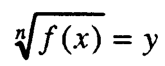# Irrational equations

An equation in which at least one of its terms is an irrational expression in relation to a variable is called an irrational equation.

The basic method for solving an irrational equation is to get rid of the roots in it, by reducing it to a rational equation (linear, quadratic, etc.).

For that purpose, we scale both sides of the equation with the same indicator. By repeating this procedure a finite number of times, the given irrational equation is reduced to a rational equation. However, it should be checked whether each root of the obtained rational equation is the root of the initial irrational equation, because when scaling with an even indicator we get an equation that is not equivalent to the given one, and when scaling with an odd indicator we get an equation that is equivalent to the initial equation.

Example. Let's solve the equation

The definition area of the equation is obtained from the terms x + Z ≥ 0 and x ≥ 0. So, D = [O, + ∞).

By separating one root from one side of the equation we get:

By squaring on both sides you get:

from where:

This equation is irrational, so by re-squaring we get x = 1.

By checking we make sure that x = 1 is a solution of the initial irrational equation.

The irrational equation of type:

with the shiftcomes down to a quadratic equation by y, i.e.

ay + by + c = 0.

The equation of type:

is resolved by the shift

###### – irrational equations –
Total Page Visits: 561 - Today Page Visits: 2